Math Geometry VocabularyPage 1

WATCH ALL SLIDES

Slide 1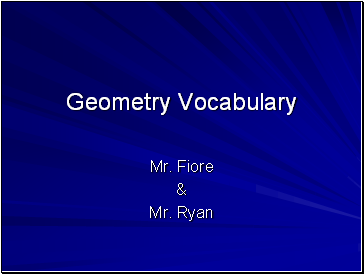Geometry Vocabulary

Mr. Fiore

&

Mr. Ryan

Slide 2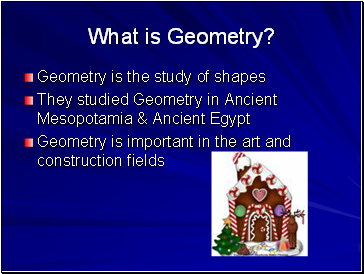What is Geometry?

Geometry is the study of shapes

They studied Geometry in Ancient Mesopotamia & Ancient Egypt

Geometry is important in the art and construction fields

Slide 3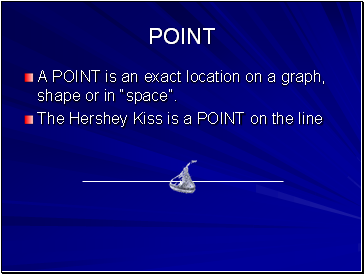Point

A POINT is an exact location on a graph, shape or in “space”.

The Hershey Kiss is a POINT on the line

Slide 4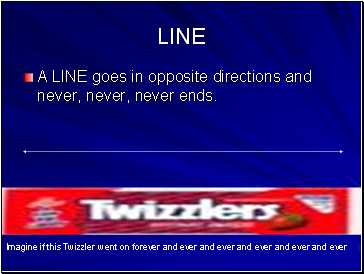Line

A LINE goes in opposite directions and never, never, never ends.

Imagine if this Twizzler went on forever and ever and ever and ever and ever and ever

Slide 5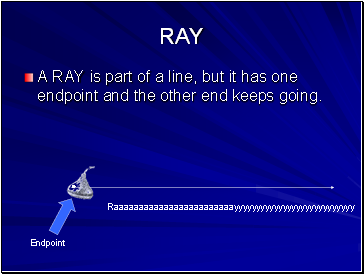Ray

A RAY is part of a line, but it has one endpoint and the other end keeps going.

Endpoint

Raaaaaaaaaaaaaaaaaaaaaaaayyyyyyyyyyyyyyyyyyyyyyyyyyy

Slide 6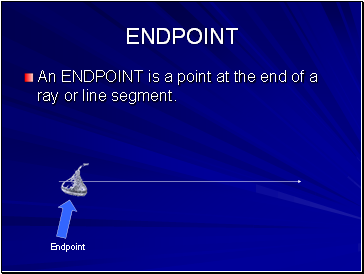Endpoint

An ENDPOINT is a point at the end of a ray or line segment.

Endpoint

Slide 7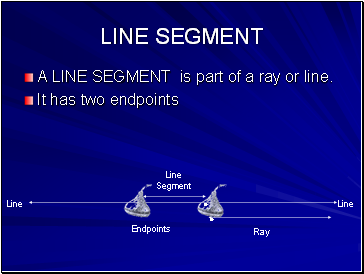Line segment

A LINE SEGMENT is part of a ray or line.

It has two endpoints

Line Segment

Line

Line

Ray

Endpoints

Slide 8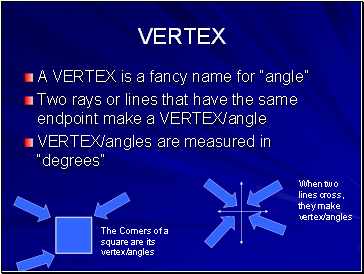Vertex

A VERTEX is a fancy name for “angle”

Two rays or lines that have the same endpoint make a VERTEX/angle

VERTEX/angles are measured in “degrees”

The Corners of a square are its vertex/angles

When two lines cross, they make vertex/angles

Slide 9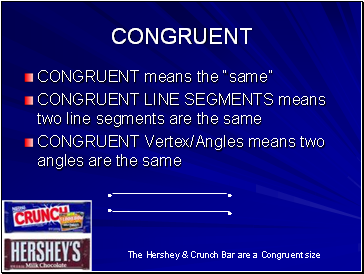Congruent

CONGRUENT means the “same”

CONGRUENT LINE SEGMENTS means two line segments are the same

CONGRUENT Vertex/Angles means two angles are the same

The Hershey & Crunch Bar are a Congruent size

Slide 10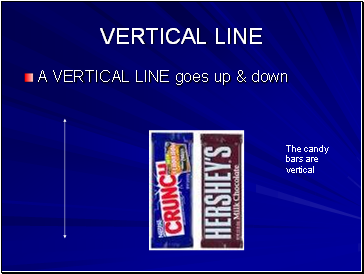Vertical line

A VERTICAL LINE goes up & down

The candy bars are vertical

Slide 11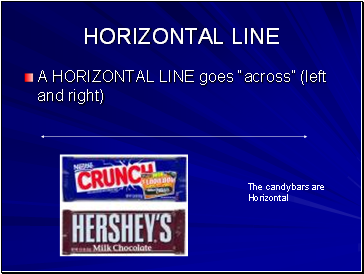Horizontal line

A HORIZONTAL LINE goes “across” (left and right)

The candy bars are Horizontal

Slide 12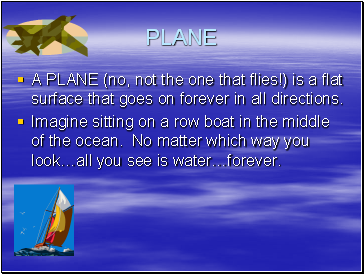Go to page:
1  2